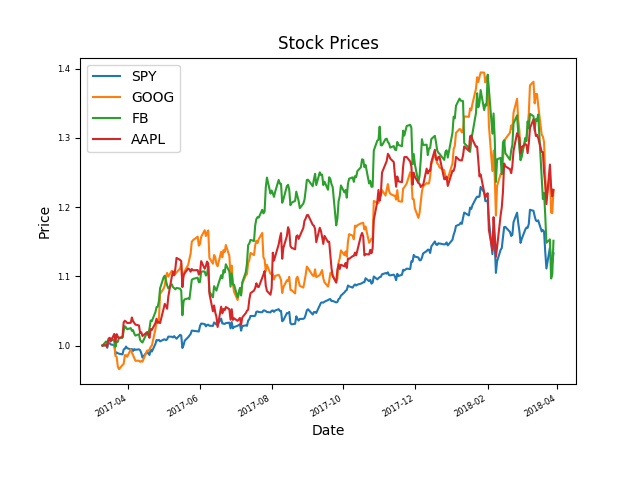# Motivation & Python Tools Stack

### Motivation

10几年过去了，虽然从事是非金融类工作，对投资还是一直很执着，学习过各种金融知识，各种市场理论，但这些知识确对提高投资水平的帮助却不大，对于投资，我觉得有句话说的特别好(忘记是谁说的了)

### 快速上手

 Date Open High Low Close Volume Adj 2008-10-14 116.26 116.40 103.14 104.08 70749800 104.08 2008-10-13 104.55 110.53 101.02 110.26 54967000 110.26 … … … … … … … 1984-09-10 26.50 26.62 25.87 26.37 2346400 3.01 1984-09-07 26.50 26.87 26.25 26.50 2981600 3.02

import pandas as pd
import matplotlib.pyplot as plt

#Get max price
max_price = df['Close'].max() # max_price:  199.83

#Get avg. volume
mean_volume = df['Volume'].mean() #avg_volume:  13639864.0684098

#Data Visualization
df['Close'].plot()
plt.show()


### Building DataFrames

import pandas as pd

start_date = '2018-03-01'
end_date = '2018-03-30'
dates = pd.date_range(start_date, end_date)
# <class 'pandas.core.indexes.datetimes.DatetimeIndex'>
#dates = 2018-03-22 00:00:00

#Create an empty dataframe
#index = date
df1 = pd.DataFrame(index=dates)
#index = "Date"
index_col="Date",
parse_dates=True,
na_values=['nan'])
#Rename Column name
#Inner Join S&P500
df1 = df1.join(dfSPY,how="inner");
symbols = ['GOOG','FB','AAPL'];
for name in symbols:
index_col="Date",
parse_dates=True,
na_values=['nan']);

#left join all tables to df1
df1 = df1.join(df_tmp) #how="left";
print(df1.ix['2018-03-01':'2018-03-06']) #first 6 days



                   SPY         GOOG          FB        AAPL
2018-03-01  265.440216  1069.520020  175.940002  173.718521
2018-03-02  266.808533  1078.920044  176.619995  174.919678
2018-03-05  269.892303  1090.930054  180.399994  175.525208
2018-03-06  270.576477  1095.060059  179.779999  175.376297


#normalized data
df1 = df1 / df1.ix[0,:]
ax = df1.plot(title='Stock Prices', fontsize=6)
ax.set_xlabel("Date")
ax.set_ylabel("Price")
plt.show()（全文完）